Next: Newton's Third Law of Up: Newton's Laws of Motion Previous: Newton's First Law of

Newton's Second Law of Motion

Newton's second law of motion essentially states that if a point object is subject to an external force,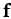, then its equation of motion is given by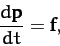(13)

where the momentum,, is the product of the object's inertial mass,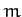, and its velocity,. Ifis not a function of time then the above expression reduces to the familiar equation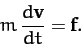(14)

Note that this equation is only valid in a inertial frame. Clearly, the inertial mass of an object measures its reluctance to deviate from its preferred state of uniform motion in a straight-line (in an inertial frame). Of course, the above equation of motion can only be solved if we have an independent expression for the force,(i.e., a law of force). Let us suppose that this is the case.

An important corollary of Newton's second law is that force is a vector quantity. This must be the case, since the law equates force to the product of a scalar (mass) and a vector (acceleration). Note that acceleration is obviously a vector because it is directly related to displacement, which is the prototype of all vectors--see Appendix A. One consequence of force being a vector is that two forces,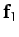and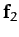, both acting at a given point, have the same effect as a single force,, acting at the same point, where the summation is performed according to the laws of vector addition--see Appendix A. Likewise, a single force,, acting at a given point, has the same effect as two forces,and, acting at the same point, provided that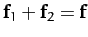. This method of combining and splitting forces is known as the resolution of forces, and lies at the heart of many calculations in Newtonian dynamics.

Taking the scalar product of Equation (14) with the velocity,, we obtain(15)

This can be written(16)

where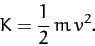(17)

The right-hand side of Equation (16) represents the rate at which the force does work on the object: i.e., the rate at which the force transfers energy to the object. The quantityrepresents the energy the object possesses by virtue of its motion. This type of energy is generally known as kinetic energy. Thus, Equation (16) states that any work done on point object by an external force goes to increase the object's kinetic energy.

Suppose that, under the action of the force,, our object moves from pointat time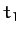to point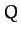at time. The net change in the object's kinetic energy is obtained by integrating Equation (16):(18)

since. Here,is an element of the object's path between pointsand, and the integral in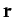represents the net work done by the force as the objects moves along the path fromto.

As described in Section A.15, there are basically two kinds of forces in nature. Firstly, those for which line integrals of the typedepend on the end points, but not on the path taken between these points. Secondly, those for which line integrals of the typedepend both on the end points, and the path taken between these points. The first kind of force is termed conservative, whereas the second kind is termed non-conservative. It was also demonstrated in Section A.15 that if the line integralis path independent then the forcecan always be written as the gradient of a scalar field. In other words, all conservative forces satisfy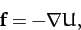(19)

for some scalar field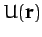. Note that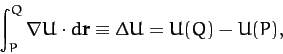(20)

irrespective of the path taken betweenand. Hence, it follows from Equation (18) that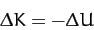(21)

for conservative forces. Another way of writing this is(22)

Of course, we recognize this as an energy conservation equation:is the object's total energy, which is conserved;is the energy the object has by virtue of its motion, otherwise know as its kinetic energy; and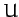is the energy the object has by virtue of its position, otherwise known as its potential energy. Note, however, that we can only write such energy conservation equations for conservative forces. Gravity is a good example of a conservative force. Non-conservative forces, on the other hand, do not conserve energy. In general, this is because of some sort of frictional energy loss which drains energy from the dynamical system whilst it remains in motion. Note that potential energy is undefined to an arbitrary additive constant. In fact, it is only the difference in potential energy between different points in space that is well-defined.Next: Newton's Third Law of Up: Newton's Laws of Motion Previous: Newton's First Law of
Richard Fitzpatrick 2011-03-31